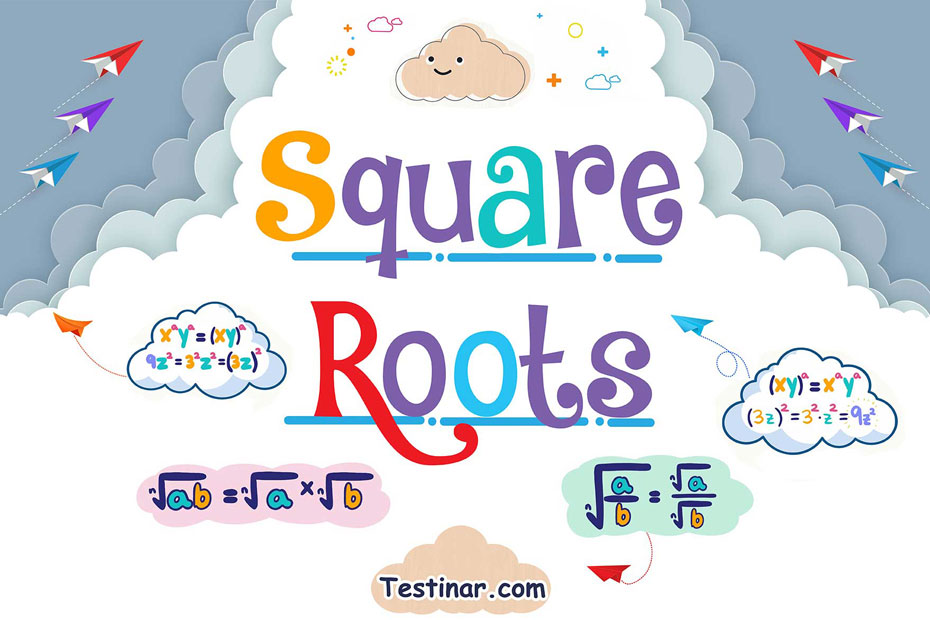## What is the Square Root of a Number

The square root of an integer is the reciprocal of the squaring process. The square of a number is equal to the value of the number's power $$2$$, whereas the square root of a number is the number that must be multiplied by itself in order to obtain the original number. If the letter '$$a$$' is the square root of the letter '$$b$$,' then follows that $$a \times a \ = \ b$$.
Because the square of any number is always a positive number, every number has two square roots, one with a positive value and one with a negative value, resulting in a total of two square roots for every number. For instance, both $$2$$ and $$-2$$ are square roots of the integer $$4$$. However, you will see that in the majority of cases, only the positive number is written as the square root.

### Methods to Find Square Roots

Finding the square root of an integer that is a perfect square is a straightforward process. Those positive numbers that may be expressed as the multiplication of a number by itself are referred to as "perfect squares." To put it another way, perfect squares are numbers that are the value of the power $$2$$ of any positive integer. Finding the square root of an integer can be accomplished in one of four ways, which are as follows:

• Repeated Subtraction
• Prime Factorization Method
• Estimation Method
• Square Root by Long Division

Remember that the first three ways are most handy for perfect squares, but the fourth approach, i.e., the long division method, may be used for any number, whether it is a perfect square or not, and is, therefore, the most general method available.

### Square Root by Repeated Subtraction

This is a relatively straightforward procedure. The square root of an integer is found by subtracting the consecutive odd numbers from the number for which the square root is sought until we reach zero. The amount of times we subtract equals the square root of the number we are working with. This approach is only effective for integers that are perfect squares. Now, let us use this method to calculate the square root of $$25$$.

• $$25 \ – \ 1 \ = \ 24$$
• $$24 \ – \ 3 \ = \ 21$$
• $$21 \ – \ 5 \ = \ 16$$
• $$16 \ – \ 7 \ = \ 9$$
• $$9 \ – \ 9 \ = \ 0$$

We have deducted $$5$$ times, as you can see from the table. As a result, the square root of $$25$$ is $$5$$.

### Square Root by Prime Factorization Method

When a number is prime factorized, it signifies that it may be represented as a product of two or more prime numbers. In order to find the square root of a given integer using the prime factorization method, we must first do the procedures outlined below:

• Subdivide the provided integer into its prime factors and multiply the result.
• Form pairs of factors that are comparable to one another, such that the two factors in each pair are equal.
• Select one of the factors from the group.
• Calculate the product of the factors obtained by selecting one component from each pair.
• The square root of the provided number is represented by this product.

So, let’s find the square root of $$144$$. Now, we know that $$144$$ can be prime factorized as $$2 \times 2 \times 2 \times 2 \times 3 \times 3.$$ So, taking $$2$$ common from the first pair, $$2$$ common from the second pair, and $$3$$ common from the third pair and multiplying them we get $$2 \times 2 \times 3 \ = \ 12$$. So, $$12$$ is the square root of $$144$$.

### Exercises for Square Roots

1) $$\sqrt{16} =$$

2) $$\sqrt{192} =$$

3) $$\sqrt{144} =$$

4) $$\sqrt{324} =$$

5) $$\sqrt{25} =$$

6) $$\sqrt{36} =$$

7) $$\sqrt{169} =$$

8) $$\sqrt{45} =$$

9) $$\sqrt{300} =$$

10) $$\sqrt{49} =$$

1) $$\sqrt{16} =$$$$\ \color{red}{4}$$
2) $$\sqrt{192} =$$$$\ \color{red}{8\sqrt{3}}$$
3) $$\sqrt{144} =$$$$\ \color{red}{12}$$
4) $$\sqrt{324} =$$$$\ \color{red}{9\sqrt{4}}$$
5) $$\sqrt{25} =$$$$\ \color{red}{5}$$
6) $$\sqrt{36} =$$$$\ \color{red}{6}$$
7) $$\sqrt{169} =$$$$\ \color{red}{13}$$
8) $$\sqrt{45} =$$$$\ \color{red}{3\sqrt{5}}$$
9) $$\sqrt{300} =$$$$\ \color{red}{10\sqrt{3}}$$
10) $$\sqrt{49} =$$$$\ \color{red}{7}$$

## Square Roots Quiz

### TASC Mathematics Formulas

$6.99$5.99

### ACT Mathematics Formulas

$6.99$5.99

### GED Math in 10 Days

$26.99$13.99

### 5 Full Length SSAT Middle Math Practice Tests

$11.99$15.99